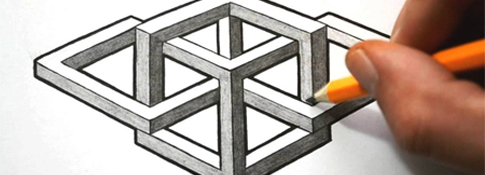##### Geometric Shapes #6###### Source: Listen in English

A simple drawing of geometric shapes including squares, circles, rectangles, triangles, vertical lines, horizonatal lines and diagonal lines.###Directions

1. REVIEW the vocabulary.
2. LISTEN to the audio above.
3. DRAW the picture (on a piece of paper).

###Vocabulary

If you don't know any of the words below, check the Illustrated Dictionary.

• a circle
• a rectangle
• a square
• a triangle
• a line
• horizontal
• vertical
• diagonal
• right-side up
• upside down

###Grammar (s-v agreement)

Directions: 1) Choose the determiner that you think best fits the sentence. 2) Listen again and check your answers.

• I (am) looking at a picture in landscape, so it (is) wider than it (is) tall.
• On the left side of the picture, there (are) three squares.
• At the top, the square (has) a diagonal line going from the top left corner to the bottom right corner.
• The next square down, the middle square, (has) a line going from the left side in the middle to the right side in the middle.
• At the bottom of the page, on the left, the third square (has) a line dividing the square into left and right.
• In the middle of the page, at the top, there (is) a circle.
• The circle (is) the same size as the squares.
• At the bottom of circle, inside, there (is) a small circle.
• It (is) very small.
• Below the big circle, there (is) a very small square.
• On the right side of the page, there (is) a rectangle going from the top of the page to the bottom.
• At the top of the rectangle, there (are) three lines.
• The lines (are) horizontal and parallel.

###Script

I am looking at a picture in landscape, so it is wider than it is tall.

On the left side of the picture, there are three squares.

At the top, the square has a diagonal line going from the top left corner to the bottom right corner.

The next square down, the middle square, has a line going from the left side in the middle to the right side in the middle.

At the bottom of the page, on the left, the third square has a line dividing the square into left and right.

In the middle of the page, at the top, there is a circle.

The circle is the same size as the squares.

At the bottom of circle, inside, there is a small circle.

It is very small.

Below the big circle, there is a very small square.

On the right side of the page, there is a rectangle going from the top of the page to the bottom.

At the top of the rectangle, there are three lines.

The lines are horizontal and parallel.